# Kendrick's MATLAB Utility Examples

##  alignvolumedata

``` % load in high-resolution volume (1 mm x 1 mm x 1 mm)
ref = getsamplebrain(3);

% load in in-plane anatomy (0.75 mm x 0.75 mm x 3 mm)
target = getsamplebrain(2);

% create an initial transformation struct.
% this transformation allows only rigid-body transformations (translations, rotations).
% the transformation is intentionally imperfect so that we can let the alignment
%   utility improve the transformation.
tr = maketransformation([0 0 0],[1 2 3],[125 59 137],[1 2 3],[100 0 0],[256 256 16],[192 192 48],[1 1 1],[0 0 0],[0 0 0],[0 0 0]);

% start up the alignment utility
alignvolumedata(ref,[1 1 1],target,[0.75 0.75 3],tr);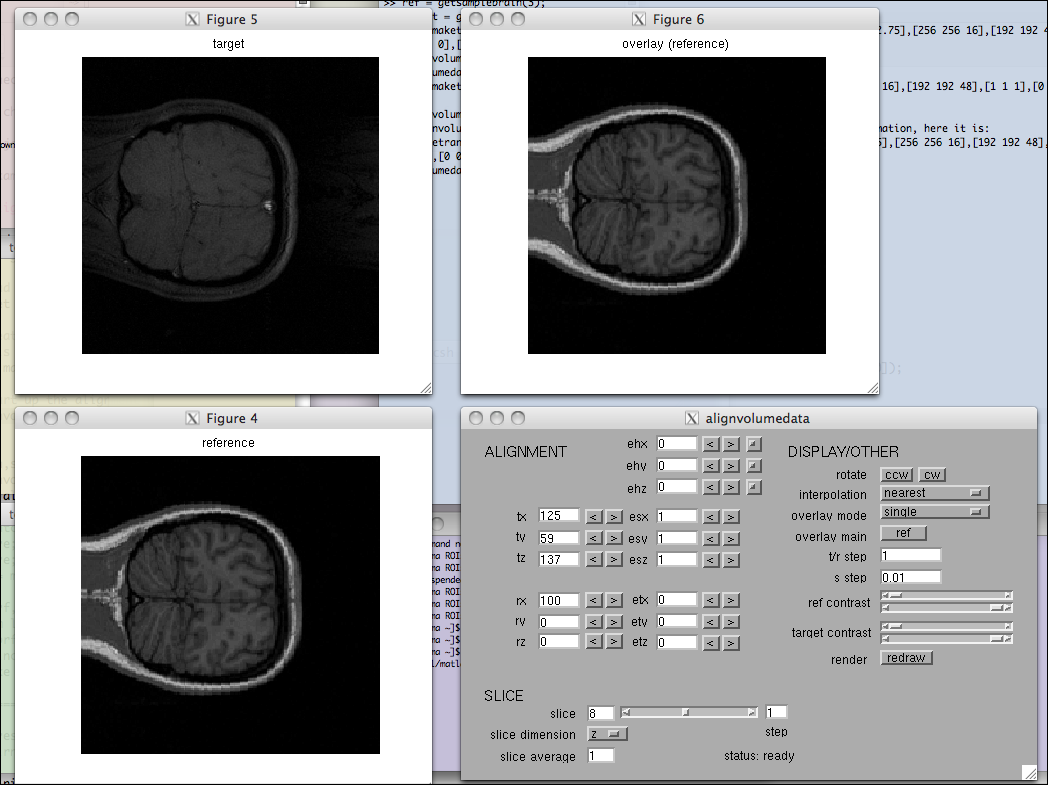% to make the visualization nicer, rotate the view CCW,
%   change to linear interpolation, and increase the contrast: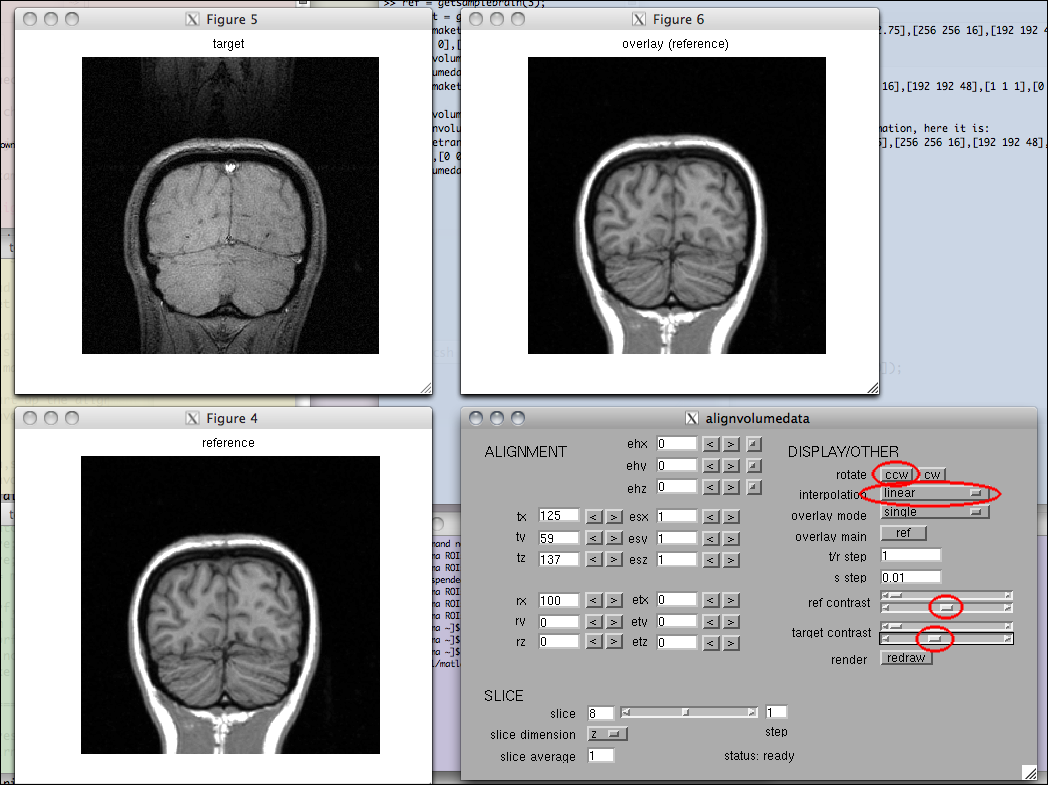% now, we need to define an ellipse on the in-plane anatomy so
% that the automated alignment routine will know what voxels to
% consider.  issue the following command:
[f,mn,sd] = defineellipse3d(target);

% the defineellipse3d command will come up with an initial seed
% for the 3D ellipse based on a model fit.  then a window with
% this initial seed will appear: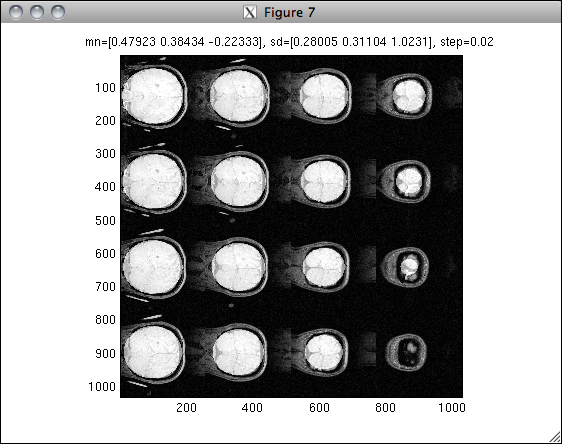% to improve the ellipse (such that nothing outside of the brain
% is included), press "i i j j j j d" in the figure window.
% the result will look like this: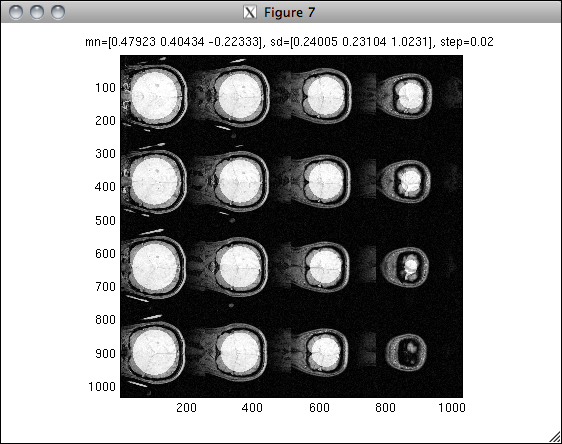% to indicate that we are done adjusting the ellipse, press ESC.
% then close the window.

% now we want to try automated alignment adjustment.  we will use
% the 3D ellipse that we have defined.  we will allow only rigid-body
% transformations, and to increase the speed of the automated alignment,
% we will optimize the transformation based on every 4 voxels in the
% first two dimensions and every 2 voxels in the third dimension:
alignvolumedata_auto(mn,sd,0,[4 4 2]);

% after issuing the above command, a window will come up with a
% visualization of the 3D ellipse that is being used (the images
% at the left show the ellipse in black; the images at the right
% depict the ellipse as a mask).  move the window aside: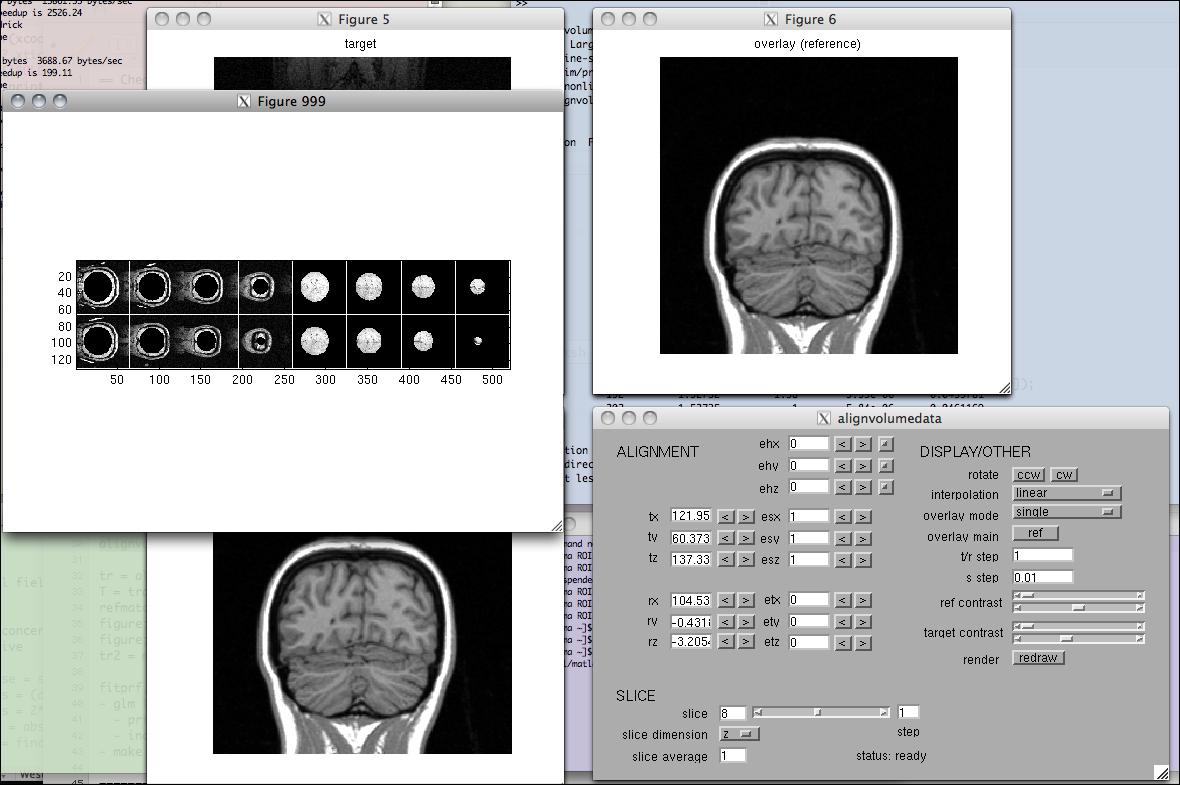% as a result of the alignvolumedata_auto command, the transformation
% will be automatically optimized.  as the optimization progresses,
% the visualization will at each iteration automatically flip a
% few times between the target volume and extracted slices through
% the reference volume.  the alignment will proceed until convergence
% is reached.  information about convergence will be printed
% to the command window: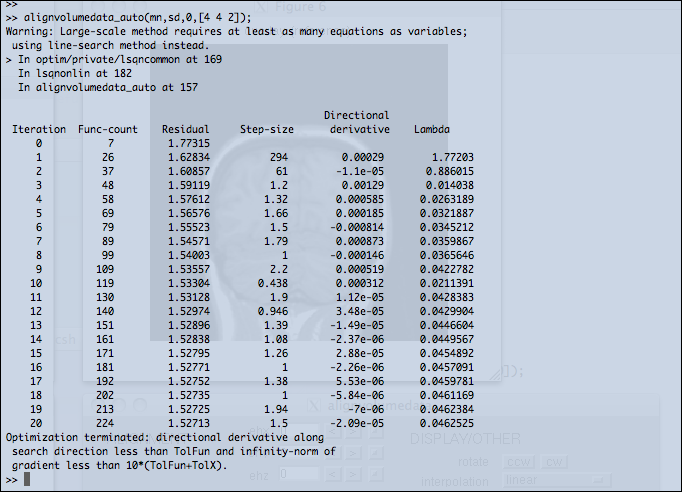% after the optimization is done, get the parameters out:
tr = alignvolumedata_exporttransformation;

% the parameters will look something like this:
tr = maketransformation([0 0 0],[1 2 3],[121.9573532348 60.3737456392266 137.333414835458],[1 2 3],[104.536550283342 -0.431811239199789 -3.20539726235996],[256 256 16],[192 192 48],[1 1 1],[0 0 0],[0 0 0],[0 0 0]);

% now, for fun, we can convert the parameters into a more standard format,
% specificcally, a 4x4 transformation matrix that maps voxels in the target volume
% to voxels in the reference volume.  note that the space assumed is 1-indexed
% matrix space --- for example, coordinates (5,3,4) in the target volume
% correspond to the center of the voxel that is at target(5,3,4).
T = transformationtomatrix(tr,0,[1 1 1])
0.748805359729198       -0.0159888393303582        -0.156710702005658          29.1224713305972
-0.04193547741802        -0.187647717107177         -2.89973722946942          114.522952586205
0.00565234239487326          0.72597017244604        -0.752971284914568          49.7201776001749
0                         0                         0                         1

% for a final sanity check, we extract slices through the reference volume
% that, if the alignment is accurate, should match the target volume.
% these slices will be the same size and dimensions as the original target volume.
refmatch = extractslices(ref,[1 1 1],target,[0.75 0.75 3],T);

% let's inspect the results:
figure; imagesc(makeimagestack(target,1)); axis equal tight;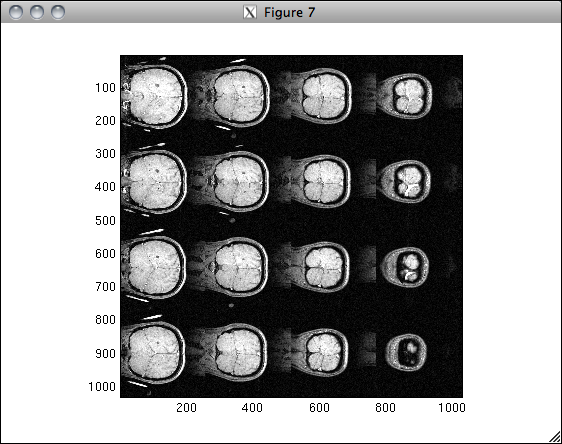figure; imagesc(makeimagestack(refmatch,1)); axis equal tight;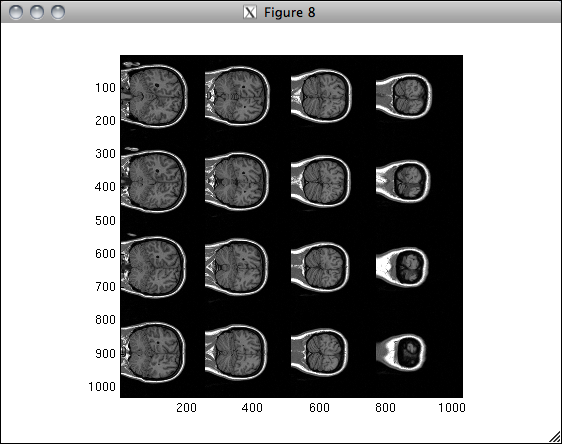```

##  fitprf (and fitprfgui) [GLM example]

``` % load in some sample data
disp(stimulus)
disp(response)
disp(tr)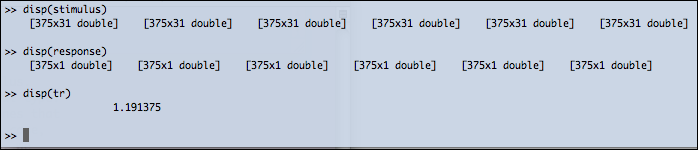% 'response' contains time-series data from one voxel.
% there are six distinct runs, each run consists of 375 volumes,
% and the TR is 1.191375 s.

% let's inspect the response.
figure; setfigurepos([.1 .1 .8 .2]); hold on;
plot(cat(1,response{:}),'r-');
straightline(cumsum(cellfun(@length,response)) + .5,'v','k-');
xlabel('TR'); ylabel('BOLD signal (arbitrary units)');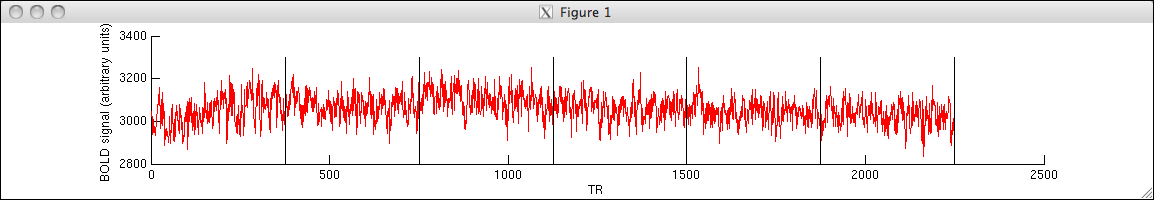% 'stimulus' contains the representation of the stimulus.
% we have 31 regressors, one regressor for each trial type.
% each regressor consists of all zeros except for ones that
% indicate the onset of a given trial type.  in our case,
% each trial type is shown twice in each run.  also, we
% periodically have null trials, the existence of which
% helps us estimate the baseline response.  each trial type
% corresponds to presentation of a 3-s visual stimulus.

% let's inspect the stimulus for the first run.
figure; setfigurepos([.1 .1 .8 .5]);
subplot(1,2,1); hold on; imagesc(stimulus{1}); axis image normal; xlabel('regressor'); ylabel('TR');
subplot(2,2,2); hold on; plot(sum(stimulus{1},1),'ro'); xlabel('regressor'); ylabel('total number of trial onsets');
subplot(2,2,4); hold on; plot(sum(stimulus{1},2)); xlabel('TR'); ylabel('trial onsets');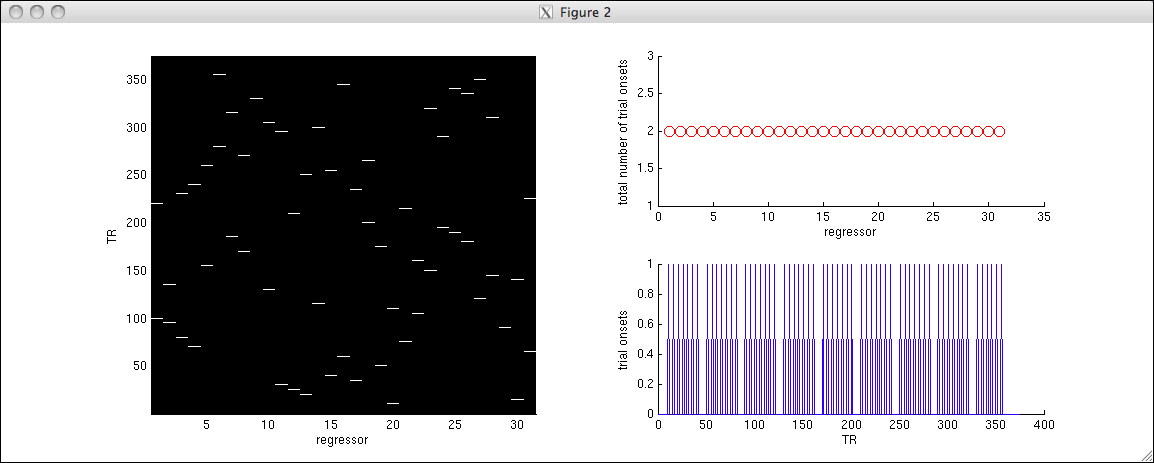% ok, we're ready to model the data using fitprf.m.
% for convenience, let's use fitprfgui.m.
fitprfgui;

% edit the fields circled in red and then click the
% "Run and Save to Workspace" button.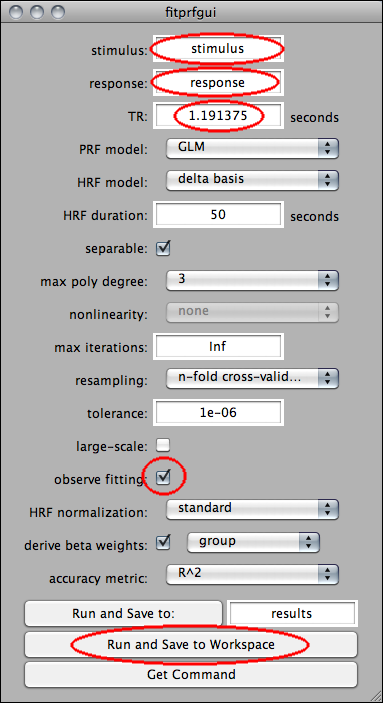% by clicking the button, a few things will occur:
% first, a command will be reported to the command window.
% this command is a call to fitprf.m that reflects the various
% values that we have specified in the GUI.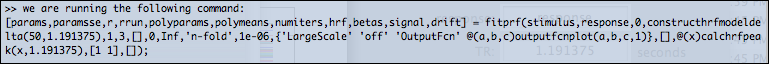% then, the command will be run.  because the resampling scheme is
% n-fold cross-validation, there will be six separate model fits.
% given that we clicked the "observe fitting" checkbox, each model fit
% will open up a figure window where the progress of model fitting
% will be shown.  here is an example: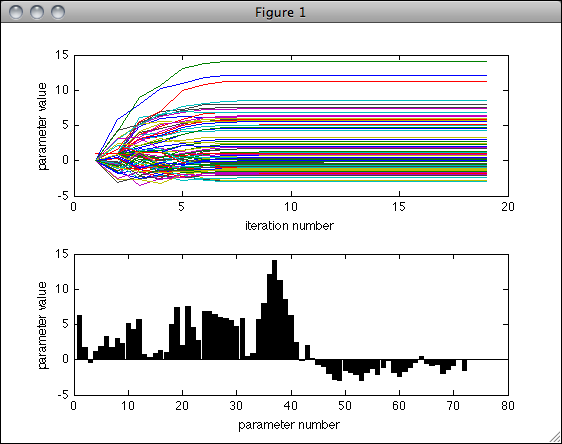% also, as the fitting progresses, various optimization information
% will be reported to the command window.  here is an example: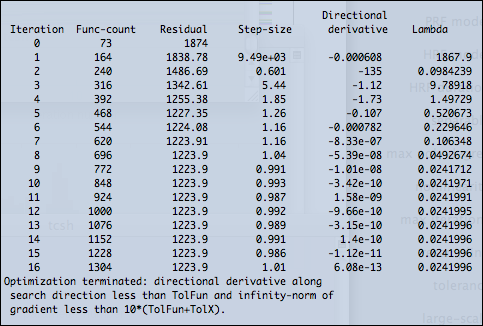% after the command is finished, a number of variables will be saved
% to the workspace.  let's look at the PRF and HRF estimates:
figure; setfigurepos([.1 .1 .5 .3]);
subplot(1,2,1); hold on;
% we want to see only the betas from the training runs, so we skip every other beta weight.
% also, to convert to percent signal change, we simply divide by the mean of all the data
% and then multiply by 100.  (please note that in general, the format of <betas> depends on the
% resampling scheme and the type of beta weight derivation (e.g. group or individual).  see
% fitprf.m for details.)
plot(betas(:,1:2:end)/mean(cat(1,response{:})) * 100,'-');
ax = axis; axis([0 size(betas,1)+1 ax(3:4)]);
straightline(0,'h','k-');
xlabel('beta number');
ylabel('BOLD signal (% change)');
title(sprintf('estimated PRF; R^2=%.1f',r));
subplot(1,2,2); hold on;
plot(0:tr:tr*(size(hrf,1)-1),hrf,'-');
ax = axis; axis([0 ceil(tr*(size(hrf,1)-1)) ax(3:4)]);
straightline(0,'h','k-');
xlabel('time from trial onset (s)');
ylabel('BOLD signal (arbitrary units)');
title(sprintf('estimated HRF; R^2=%.1f',r));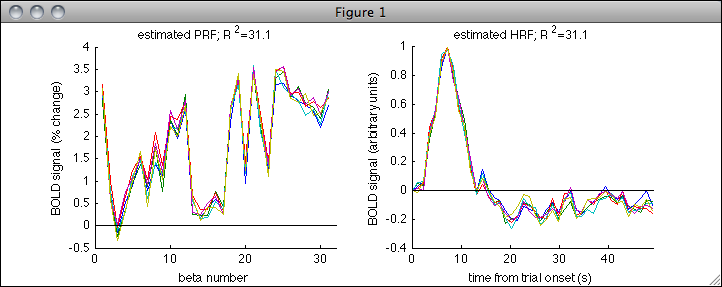% the left panel of the figure shows the beta weight corresponding to
% each of the 31 trial types.  the beta weights have been converted to
% percent signal change.  there are six traces, one trace for each
% model fit.  what we are viewing is actually the beta weight derived
% from analyzing all of the training runs as a group.  for example,
% the first model fit involves training on runs 2-6 and testing on run 1,
% and what we are plotting here are the beta weights estimated from
% analyzing runs 2-6.  the variability of the traces reflects measurement
% noise.  (in fact, n-fold cross-validation is identical to the jackknife
% technique, and we could obtain an estimate of standard error by taking
% the standard deviation across the six traces and scaling by the square
% root of one minus the number of jackknifes (in this case, sqrt(6-1)).)
%
% the right panel of the figure shows the estimated HRF for each model fit.
% in the GUI, we specified a delta-basis HRF model that extends up to 50 seconds
% after the trial onset.  this model includes a free parameter for every time point
% in the HRF except for the first time point (which is coincident with the
% very beginning of the trial) which is constrained to be always zero.
% because we selected standard HRF normalization in the GUI, all HRF
% estimates are normalized to have a peak of 1.  there are six traces,
% one trace for each model fit.  the variability of the traces reflects
% measurement noise.

% finally, for fun, let's look at how well the model fits the time-series data.
% (please note that in general, the format of <signal> and <drift> depends on
% the resampling scheme; see fitprf.m for details.)
figure; setfigurepos([.1 .1 .6 .2]); hold on;
% look at the training runs used for the first model fit
plot(cat(1,response{2:end}),'r-');
% look at the estimated drift for the training runs in the first model fit
plot(drift(1:375*5),'g-');
% look at the estimated drift plus the estimated signal for the training runs in the first model fit
plot(signal(1:375*5)+drift(1:375*5),'k-');
% let's look at a small range of the data
ax = axis; axis([400 700 ax(3:4)]);
xlabel('TR'); ylabel('BOLD signal (arbitrary units)');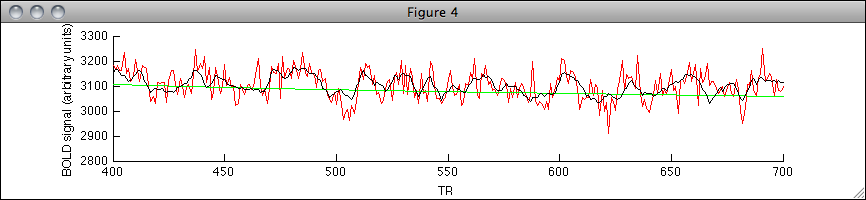% the red trace indicates the raw data.  the green trace indicates the
% estimated drift (which is modeled using a weighted sum of polynomials up to
% degree 3, as specified in the GUI).  the black trace indicates the
% estimated drift plus the estimated signal (i.e. the component of the data
% due to the PRF and HRF).
```

##  fitprf (and fitprfgui) [retinotopic mapping example]

``` % load in some sample data
disp(stimulus)
disp(response)
disp(tr)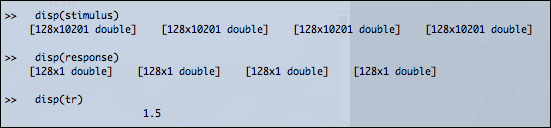% 'response' contains time-series data from one voxel.
% there are four distinct runs, each run consists of 128 volumes,
% and the TR is 1.5 s.

% let's inspect the response.
figure; setfigurepos([.1 .1 .8 .2]); hold on;
plot(cat(1,response{:}),'r-');
straightline(cumsum(cellfun(@length,response)) + .5,'v','k-');
xlabel('TR'); ylabel('BOLD signal (arbitrary units)');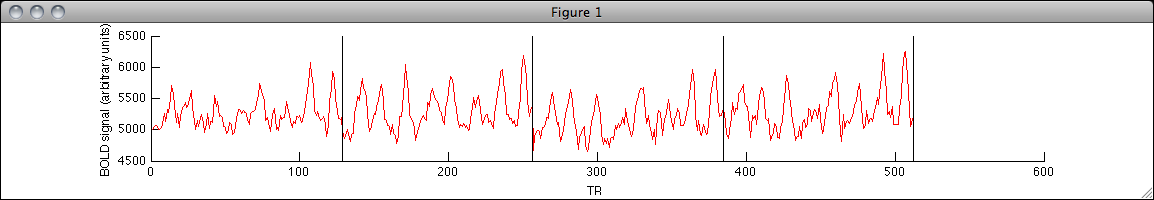% 'stimulus' contains the representation of the stimulus.
% at each time point, we have a 101 x 101 image consisting
% of 0s and 1s where 1s indicate the location of a contrast
% pattern in the visual field.  note that the stimulus is
% "flattened", with dimensions 128 x 101*101 for each run.
% also, note that the stimulus is in fact identical across
% the four runs, but there is no reason that this must
% be the case.

% let's inspect the stimulus at the first time point.
figure; setfigurepos([.1 .1 .5 .5]); hold on;
imagesc(reshape(stimulus{1}(1,:),[101 101])); axis equal tight ij;
xlabel('x'); ylabel('y');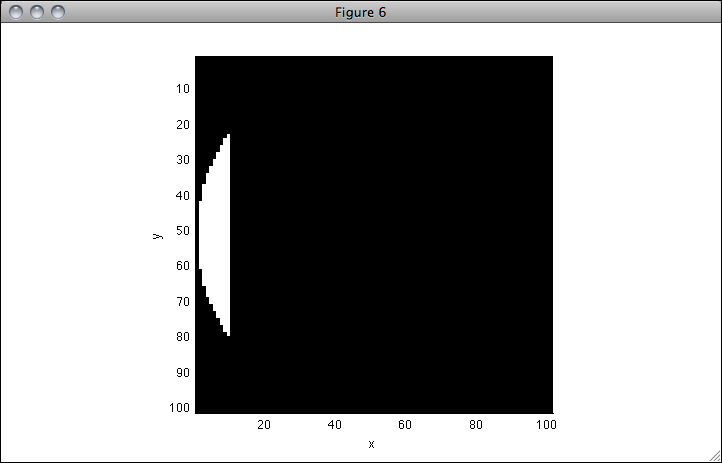% ok, we're ready to model the data using fitprf.m.
% for convenience, let's use fitprfgui.m.
fitprfgui;

% edit the fields circled in red and then click the
% "Run and Save to Workspace" button.  note that we
% click the large-scale checkbox because the PRF model
% involves boundary constraints.  (if you neglect to
% click the checkbox, the worst that will happen is
% that the optimizer will issue a warning and switch on
% the large-scale option for you.)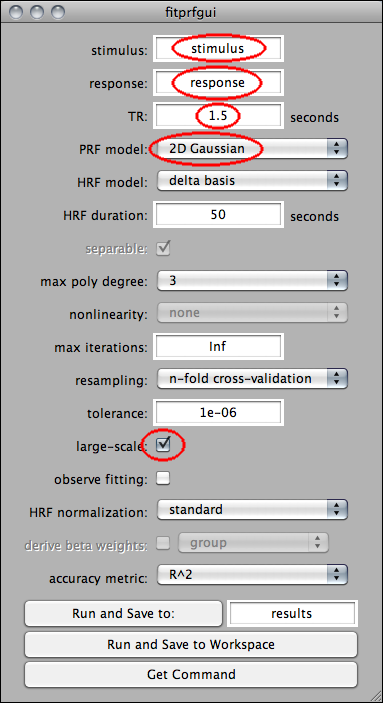% since we are using n-fold cross-validation, four separate
% model fits will be performed.  since we did not check the
% "observe fitting" checkbox, no figure windows will be
% opened.  however, optimization information will still be
% reported to the command window.  at the end of the fitting,
% a number of variables will be saved to the workspace.

% let's look at the PRF and HRF estimates:
figure; setfigurepos([.1 .1 .5 .3]);
subplot(1,2,1); hold on;
for p=1:size(params,1)
% note that we visualize the contour at +/- 2 std devs
h = drawellipse(params(p,1),params(p,2),0,2*params(p,3),2*params(p,3));
set(h,'Color',rand(1,3));
end
axis equal; axis([.5 101.5 .5 101.5]); axis ij;
xlabel('x-position'); ylabel('y-position');
title(sprintf('estimated PRF; R^2=%.1f',r));
subplot(1,2,2); hold on;
plot(0:tr:tr*(size(hrf,1)-1),hrf,'-');
ax = axis; axis([0 ceil(tr*(size(hrf,1)-1)) ax(3:4)]);
straightline(0,'h','k-');
xlabel('time from trial onset (s)');
ylabel('BOLD signal (arbitrary units)');
title(sprintf('estimated HRF; R^2=%.1f',r));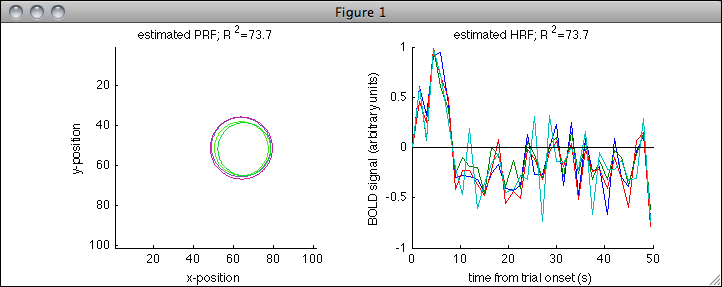% the left panel of the figure shows response contours of the fitted PRF
% models.  these contours are drawn at 2 standard deviations away from the
% peak of the Gaussian function.  there are four traces, one trace for
% each model fit.  the variability of the traces reflects measurement
% noise.  the right panel shows HRF estimates just like in the GLM example.
% notice that the HRF estimates are fairly noisy and that a more constrained
% HRF model may result in better HRF estimates.  also, note that each HRF
% estimate represents the estimated response to a single stimulus frame,
% which in our case represents 1.5 s of visual stimulation.

% for fun, let's try something quite different.
% edit the fields in the GUI as follows: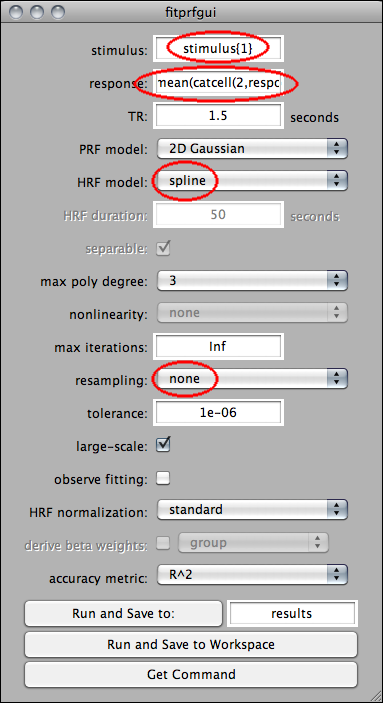% the contents of the "response" text box is:
%   mean(catcell(2,response),2)

% in this example, we are averaging the data across the four runs
% and using just a single copy of the stimulus.  this averaging
% is possible because the stimulus is identical across the four runs.
% we have also changed the HRF model to "spline" and have
% changed the resampling scheme to "none" (we cannot cross-validate
% nor bootstrap since we in effect have only one run).
% click the "Run and Save to Workspace" button.

% after the fitting is complete, inspect the PRF and HRF estimates
% (this is the same chunk of code that we ran earlier):
figure; setfigurepos([.1 .1 .5 .3]);
subplot(1,2,1); hold on;
for p=1:size(params,1)
% note that we visualize the contour at +/- 2 std devs
h = drawellipse(params(p,1),params(p,2),0,2*params(p,3),2*params(p,3));
set(h,'Color',rand(1,3));
end
axis equal; axis([.5 101.5 .5 101.5]); axis ij;
xlabel('x-position'); ylabel('y-position');
title(sprintf('estimated PRF; R^2=%.1f',r));
subplot(1,2,2); hold on;
plot(0:tr:tr*(size(hrf,1)-1),hrf,'-');
ax = axis; axis([0 ceil(tr*(size(hrf,1)-1)) ax(3:4)]);
straightline(0,'h','k-');
xlabel('time from trial onset (s)');
ylabel('BOLD signal (arbitrary units)');
title(sprintf('estimated HRF; R^2=%.1f',r));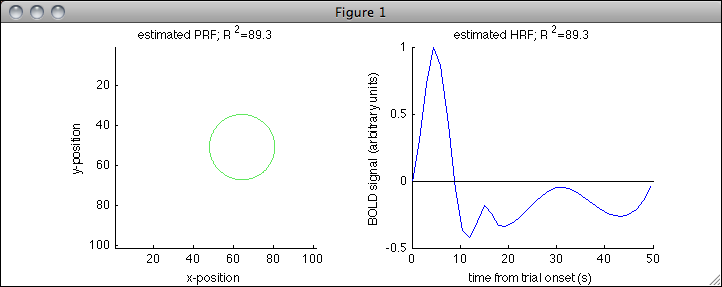% there is only one trace since we performed only one
% model fit.  also, note that the R^2 in this case
% indicates how well the model explains the
% averaged-across-runs data and, in particular,
% is not cross-validated.

% finally, for fun, let's visualize how well the model fits the time-series data
% (this figure is similar to the earlier figure we made for the GLM example):
figure; setfigurepos([.1 .1 .6 .2]); hold on;
plot(mean(catcell(2,response),2),'r-');
plot(drift,'g-');
plot(signal+drift,'k-');
xlabel('TR'); ylabel('BOLD signal (arbitrary units)');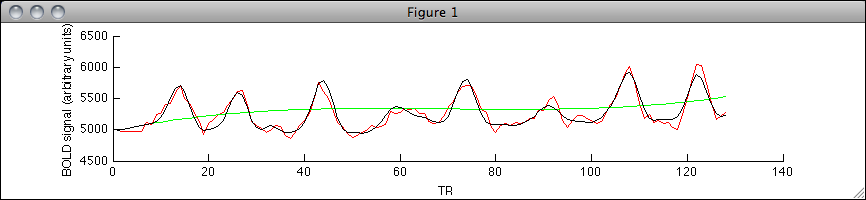```